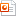검색어 입력폼

인덕터와 커패시터의 직렬 병렬

저작시기 2011.10 |등록일 2011.11.06 | 최종수정일 2017.10.11MS 파워포인트 (pptx) | 12페이지 | 가격 1,000원

소개글

RL회로의 특성
RC회로의 특성
RLC 회로의 직렬 병렬 회로의 특성 및 공진특성.

인덕터와 커패시터의 직렬 병렬

1. 실험목표

2. 관련이론

3. 실험과정

4. 점검문제

5. 조별설계문제

본문내용

1. 실험목표
(1) When inductors(L) are connected in series or parallel, Synthetic inductance value can be calculated.
(2) When Capacitors(C) are connected in series or parallel, Synthetic Capacitance value can be calculated.
(3) That can be applied to the maximum allowable current in the inductor can be understood.
(4) That can be applied to the maximum allowable current in the Capacitor can be understood.
2. 관련이론
1. The basic ingredient of the electric circuit
Inductors and inductance.
- Inductor : The coil which induces the voltage to be proportionate in change quantity of electric current
- Inductance : In compliance with the change of the electric current which flows a circuit, The electromagnetic induction furnace the pretense where it shows the ratio of the counter electromotive force which happens. The unit is the H.
(2) Capacitors and capacitance.
- Capacitor : Mainly it is a system which collects an electric charge
from electronic circuit. It does usual 2 chapter gold plates with
the electrode and with the structure which between that puts in
the insulator(dielectric) it makes.

없음